Scripting

# How to Square a Number in Python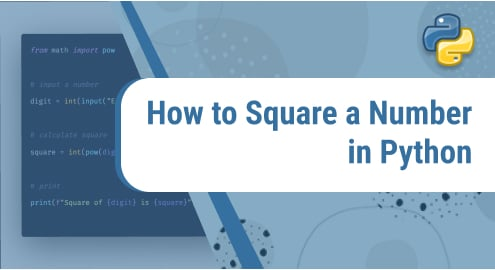Squaring a number means multiplying a number by itself. There are three ways to find the square of a number. Let’s discuss them.

1. Using multiplication
2. Using the exponent operator
3. Using the power function

## Using multiplication

One of the easiest methods in which we use the multiplication operator.

### Example:

```a= int(input("enter a number"))

print("The square of a number is", a*a)```

Output:

enter a number 45

The square of a number is 2025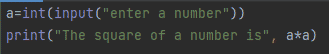Output: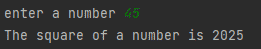In example 1, we are taking a number as input from the user and then multiplying the number by the number itself. The output of the squared number is shown in figure2.

To increase the reusability we can write it as a user-defined function.

### Example 2:

```def sqr(x):

print("The square of", x, "number is", x * x)

sqr(30)

sqr(-66)

sqr(0.75)```

Output:

The square of 30 number is 900

The square of -66 number is 4356

The square of 0.75 number is 0.5625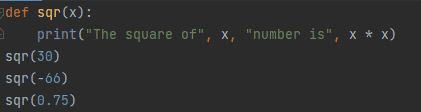Output: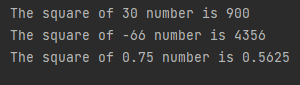In example 2 we have defined an sqr(x) function that is printing the output. The function takes one parameter which is a number. A function is called with a positive integer, negative integer, and float and we get the output accordingly, as shown in figure 4.

### Example 3:

```def sqr(x):

return x * x

print("The square of number 30 is", sqr(30))

print("The square of number -22 is", sqr(-22))

print("The square of number 6.4 is", sqr(6.4))```

Output:

The square of number 30 is 900

The square of number -22 is 484

The square of number 6.4 is 40.96000000000001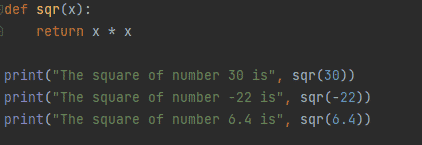Output: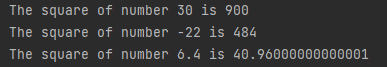In example 3 we have defined a function sqr(x) that is returning a squared number. This function takes one parameter, which is a number, and the return keyword returns the calculated value, which can be used for further calculations.

## Using Exponent operator

The second method to get a square is by using the exponent operator (**). For the exponent operator, we need two values: one is base and the second is power. To find a square we use the value of power 2 which is fixed.

### Example 1:

```a=int(input("enter a number"))

print("The square of a number is", a**2)```

Output:

enter a number 9

The square of a number is 81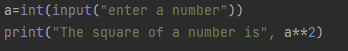Output: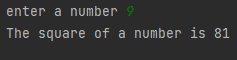In example 1, we are inputting a number from the user, which will become the base for the exponent operator, and the power is 2, which is fixed in our case. The output of the number is shown in figure 8.

We can define it as a function that returns the squared number.

### Example 2:

```def sqr_number(a):

return a ** 2

print(sqr_number(16))

print(sqr_number(3.3))

print(sqr_number(-8))```

Output:

256

10.889999999999999

64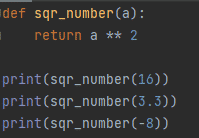Output: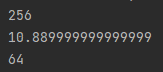In example 2, we have defined the function sqr_number(a). It takes one parameter and returns the output as shown in figure 10.

## Using power function

A power function is a built-in number in Python. It takes two parameters: base and power. To calculate power value is 2.

### Example 1:

```a=int(input("enter a number"))

print("The square of a number is", pow(a,2))```

Output:

enter a number 5

The square of a number is 25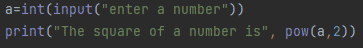Output: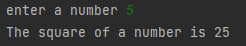In example 1, we take input from the user and calculate the square of the number using the power function. We get output as shown in figure 12.